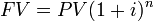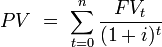## Future Value, Multiple Flows

To find the FV of multiple cash flows, sum the FV of each cash flow.

### Learning Objectives

Calculate the Future Value of Multiple Annuities

### Key Takeaways

#### Key Points

• The FV of multiple cash flows is the sum of the FV of each cash flow.
• To sum the FV of each cash flow, each must be calculated to the same point in the future.
• If the multiple cash flows are a fixed size, occur at regular intervals, and earn a constant interest rate, it is an annuity. There are formulas for calculating the FV of an annuity.

#### Key Terms

• cash flow: The sum of cash revenues and expenditures over a period of time.
• annuity: A specified income payable at stated intervals for a fixed or a contingent period, often for the recipient’s life, in consideration of a stipulated premium paid either in prior installment payments or in a single payment. For example, a retirement annuity paid to a public officer following his or her retirement.
• incremental cash flows: the additional money flowing in or out of a business due to a project

### Future Value, Multiple Cash Flows

Finding the future value (FV) of multiple cash flows means that there are more than one payment/ investment, and a business wants to find the total FV at a certain point in time. These payments can have varying sizes, occur at varying times, and earn varying interest rates, but they all have a certain value at a specific time in the future.

The first step in finding the FV of multiple cash flows is to define when the future is. Once that is done, you can determine the FV of each cash flow using the formula in. Then, simply add all of the future values together.FV of a single payment: The FV of multiple cash flows is the sum of the future values of each cash flow.

Manually calculating the FV of each cash flow and then summing them together can be a tedious process. If the cash flows are irregular, don’t happen at regular intervals, or earn different interest rates, there isn’t a special way to find the total FV.

However, if the cash flows do happen at regular intervals, are a fixed size, and earn a uniform interest rate, there is an easier way to find the total FV. Investments that have these three traits are called “annuities. ”

There are formulas to find the FV of an annuity depending on some characteristics, such as whether the payments occur at the beginning or end of each period. There is a module that goes through exactly how to calculate the FV of annuities.

If the multiple cash flows are a part of an annuity, you’re in luck; there is a simple way to find the FV. If the cash flows aren’t uniform, don’t occur at fixed intervals, or earn different interest rates, the only way to find the FV is do find the FV of each cash flow and then add them together.

## Present Value, Multiple Flows

The PV of multiple cash flows is simply the sum of the present values of each individual cash flow.

### Learning Objectives

Calculate the present value of an investment portfolio that has multiple cash flows

### Key Takeaways

#### Key Points

• To find the PV of multiple cash flows, each cash flow much be discounted to a specific point in time and then added to the others.
• To discount annuities to a time prior to their start date, they must be discounted to the start date, and then discounted to the present as a single cash flow.
• Multiple cash flow investments that are not annuities unfortunately cannot be discounted by any other method but by discounting each cash flow and summing them together.

#### Key Terms

• discount: To find the value of a sum of money at some earlier point in time. To find the present value.
• net present value: the present value of a project or an investment decision determined by summing the discounted incoming and outgoing future cash flows resulting from the decision

The PV of multiple cash flows follows the same logic as the FV of multiple cash flows. The PV of multiple cash flows is simply the sum of the present values of each individual cash flow.Sum FV: The PV of an investment is the sum of the present values of all its payments.

Each cash flow must be discounted to the same point in time. For example, you cannot sum the PV of two loans at the beginning of the loans if one starts in 2012 and one starts in 2014. If you want to find the PV in 2012, you need to discount the second loan an additional two years, even though it doesn’t start until 2014.

The calculations get markedly simpler if the cash flows make up an annuity. In order to be an annuity (and use the formulas explained in the annuity module), the cash flows need to have three traits:

1. Constant payment size
2. Payments occur at fixed intervals
3. A constant interest rate

Things may get slightly messy if there are multiple annuities, and you need to discount them to a date before the beginning of the payments.

Suppose there are two sets of cash flows which you determine are both annuities. The first extends from 1/1/14 to 1/1/16, and the second extends from 1/1/15 to 1/1/17. You want to find the total PV of all the cash flows on 1/1/13.

The annuity formulas are good for determining the PV at the date of the inception of the annuity. That means that it’s not enough to simply plug in the payment size, interest rate, and number of periods between 1/1/13 and the end of the annuities. If you do, that supposes that both annuities begin on 1/1/13, but neither do. Instead, you have to first find the PV of the first annuity on 1/1/14 and the second on 1/1/15 because that’s when the annuities begin.

You now have two present values, but both are still in the future. You then can discount those present values as if they were single sums to 1/1/13.

Unfortunately, if the cash flows do not fit the characteristics of an annuity, there isn’t a simple way to find the PV of multiple cash flows: each cash flow much be discounted and then all of the PVs must be summed together.

### Example

A corporation must decide whether to introduce a new product line. The new product will have start-up expenditures, operational expenditures, and then it will have associated incoming cash receipts ( sales ) and disbursements (Cash paid for materials, supplies, direct labor, maintenance, repairs, and direct overhead) over 12 years. This project will have an immediate (t=0) cash outflow of 100,000 (which might include all cash paid for the machinery, transportation-in and set-up expenditures, and initial employee training disbursements. ) The annual net cash flow (receipts less disbursements) from this new line for years 1-12 is forecast as follows: -54672, -39161, 3054, 7128, 25927, 28838, 46088, 77076, 46726, 76852, 132332, 166047, reflecting two years of running deficits as experience and sales are built up, with net cash receipts forecast positive after that. At the end of the 12 years it’s estimated that the entire line becomes obsolete and its scrap value just covers all the removal and disposal expenditures. All values are after-tax, and the required rate of return is given to be 10%. (This also makes the simplifying assumption that the net cash received or paid is lumped into a single transaction occurring on the last day of each year. )

The present value (PV) can be calculated for each year:

T=0: $\frac{-100,000}{(1+0.10)^0}=100,000$

T=1: $\frac{-54672}{(1+0.10)^1}=-49701.81818$

T=2: $\frac{-39161}{(1+0.10)^2}=-32364.46281$

T=3: $\frac{3054}{(1+0.10)^3}=2294.515402$

T=4: $\frac{7128}{(1+0.10)^4}=4868.51991$

T=5: $\frac{25927}{(1+0.10)^5}=16098.62714$

T=6: $\frac{28838}{(1+0.10)^6}=16278.29919$

T=7: $\frac{46088}{(1+0.10)^7}=23650.43135$

T=8: $\frac{77076}{(1+0.10)^8}=35956.52284$

T=9: $\frac{46726}{(1+0.10)^9}=19816.38532$

T=10: $\frac{76852}{(1+0.10)^{10}}=29629.77288$

T=11: $\frac{132332}{(1+0.10)^{11}}=46381.55871$

T=12: $\frac{166047}{(1+0.10)^{12}}=52907.69139$

The sum of all these present values is the net present value, which equals 65,816.04. Since the NPV is greater than zero, it would be better to invest in the project than to do nothing, and the corporation should invest in this project if there is no alternative with a higher NPV.